# Precalculus : Express a Quadratic Function in Vertex Form

## Example Questions

### Example Question #1 : Express A Quadratic Function In Vertex Form

Which of the following is the given quadratic equation in vertex form?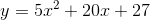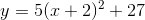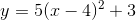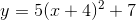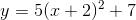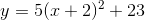Explanation:

To solve for the vertex form, we must start by completing the square: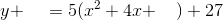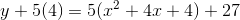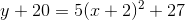### Example Question #2 : Express A Quadratic Function In Vertex Form

Which of the following is the appropriate vertex form of the following quadratic equation?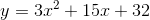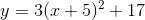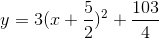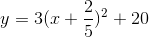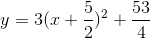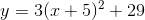Explanation:

This process outlines how to convert a quadratic function to vertex form: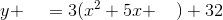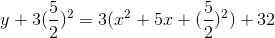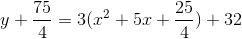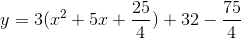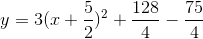### Example Question #3 : Express A Quadratic Function In Vertex Form

Give the coordinate pair of the vertex of this quadratic function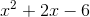.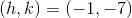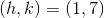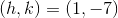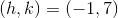Explanation:

Expressing quadratic functions in the vertex form is basically just changing the format of the equation to give us different information, namely the vertex. In order for us to change the function into this format we must have it in standard form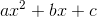. After that, our goal is to change the function into the form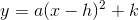. We do so as follows: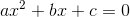subtract the constant over to the other side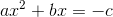halve the b term, square it, and add to both sides.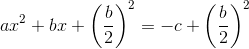Now factor the left side.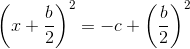now simplify the right side and move that number back over to the left side and you will be left with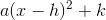. I recommend looking up an example with numbers before you begin or at least recognizing that the fractions will end up being whole numbers in most problems. Below is specific explanation of the problem at hand. Try to use the generic equation to find the answer before following the step by step approach below.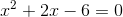move the constant over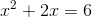halve the b term and add to both sides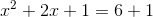factor the left side and simplify the right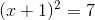move the constant over to achieve vertex form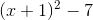is the final answer with vertex at (-1,-7). Note that the formula is.

try this shortcut after you have mastered the steps: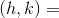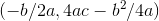. Make sure you recognize that this formula gives you an x and y coordinate for the vertex and that each coordinate of the pair is fraction in the formula. This will give you the vertex of the equation if it is in standard form. However, don't rely on this as completing the square is also a method for finding the roots. So you need to know both methods before you cut the corner.

### Example Question #1 : Express A Quadratic Function In Vertex Form

Express the following quadratic equation in vertex form.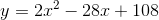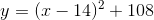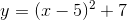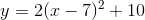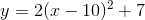Explanation:

To get the equation into vertex form, we factor the largest constant from the terms with a degree ofgreater than or equal to 1.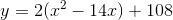We then complete the square by following these steps

1. finding half of the coefficients of theterm
2. squaring that result
3. and then adding that square to the expression for.

Keep in mind that what is done on one side of the equation must be done on the other.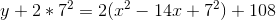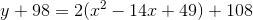And factoring the quadratic polynomial of x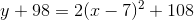we get### All Precalculus Resources